# (Q-Learning and Deep Q-Learning)

A quick note before we start. If there are any terms that are new or confusing to you, I highly recommend taking the time to Google them so that you will be able to understand the content at a much deeper level

In the future, Artificial Intelligence will be omnipresent. In 2019 spending on AI reached around \$37 billion. According to the International Data Corporation(IDC), spending on AI systems will reach \$97.9 billion by 2023. One of the growing subfields in AI that I find fascinating is Reinforcement Learning. Right now, Reinforcement is used for the creation of self-driving cars, advanced robotics, and the possibility of AI to beat humans at various games, like Chess and Go. Reinforcement Learning is also bringing us one step closer to Artificial General Intelligence, one of the coolest parts of AI.

This article will go over the basics of Reinforcement Learning, and more specifically, Q-Learning and Deep Q-Learning!

## Some Quick Vocabulary

Before we get into the basics of Reinforcement Learning, you should know some common vocabulary:

• The Agent: The Agent is the Al algorithm that you are creating; it improves over time-based on positive and negative rewards
• Environment: The Environment is the setting that the agent is in. For example, if you are playing the Pacman video game, it would be the maze with all the dots and enemies
• Policy(P): The Policy is how the agent determines what action it will take based on the current state. The objective of the Policy is to execute the best possible actions from start to finish
• Reward(R): The Reward is given to the agent based on what action it has taken in the environment
• Action(A): The Action is all the possible things that the agent can do in the environment. For example, moving left and right would be considered actions
• Q-Value(Q): The amount that an action is worth with respect to the state. The higher the Q-value is, the better

## Another Key Thing You Must Understand Is Exploration Versus Exploitation

Exploration is the amount that the agent values searching for new information in the environment. In contrast, exploitation is the degree to which the agent uses already known information to optimize the reward. These both play a significant role in finding the best policy.

# The Basics Of Reinforcement Learning

Reinforcement learning is the trial and error of Artificial Intelligence. It uses an agent in an environment to simulate what you want the algorithm to accomplish. This can be used in video games or real-life applications. Every time the agent performs an action, it gets rewarded. The environment sends this reward and a new state to the agent to remember what action gives it positive and negative rewards. The goal of the agent is to maximize its total reward.

For a funny example of Reinforcement Learning, check out this video. In this case, the Spider-Man gummies would be the reward:)

## Markov’s Decision Process

The math behind Reinforcement Learning is called Markov’s Decision Process (MDP). It uses an agent that takes action in the environment, then returns a reward and a new state. This is repeated until the agent has found the optimal policy that achieves the highest possible Q-value. The way that we can solve for MDP is through Bellman’s Equation.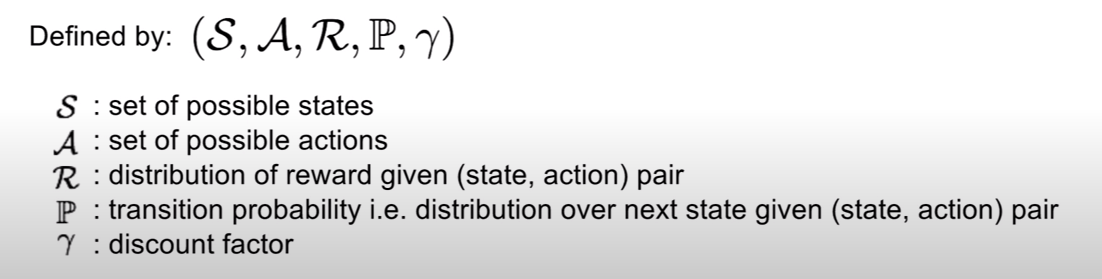Credit: Stanford Lecture 14 | Deep Reinforcement Learning

## Dynamic Programming

In 1954, Dr. Richard Bellman created a paper known as The Theory Of Dynamic Programming. The basis of Dynamic Programming is to simplify complex problems by breaking them up into smaller sub-problems. These sub-problems have to be solved recursively, which means that the function should call itself. This has been represented in the form of Bellman’s Equation.

## Bellman’s Equation

The whole point of Bellman’s Equation is to determine each state's value assuming the agent takes the best possible actions from now until the game is over. In other words, what will be the long-term reward?

# Q-Learning

The agent remembers what actions give it positive or negative values through a combination of matrices: The Reward Matrix and the Q matrix.

The Reward Matrix is a table of values that shows how much reward each action will give to the agent based on what state the agent is in.

Now, this may seem a little confusing at first; however, let me give an example. Say you are a toddler, and you are learning how to ride your bike. You can be in 6 different states. Each state corresponds to how well you are doing. If you are in state 5, you have made it to riding on your own. If you are in state 4, you have managed to ride the bike with your parent's help. If you are in State 1, you have managed to ride the bike with just training wheels. State 3 is riding your bike with human assistance and training wheels. And state 2 and 0 are the two start states. One of them has training wheels, and the other doesn’t. All the -1s represent where you cannot go. For example, you can’t get from state 0 to 5 in one step.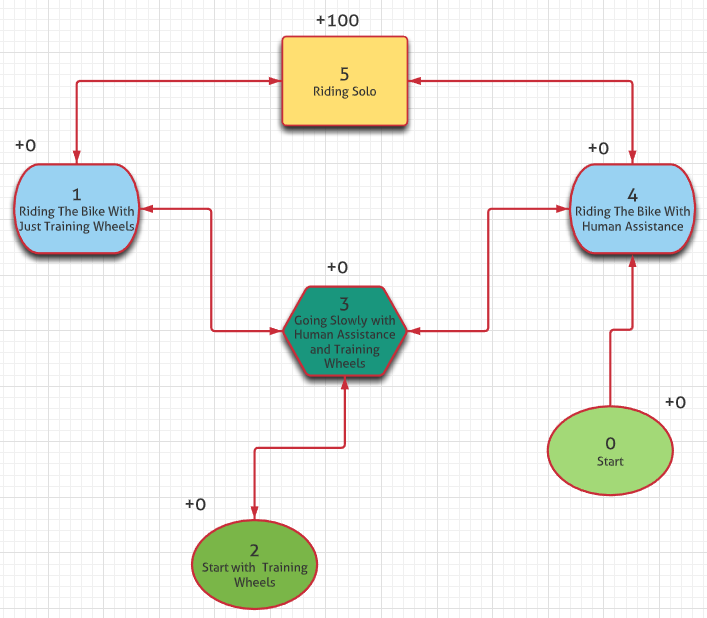Mind Map Showing Reward For each Stage In Riding A Bike

In this mind map above, you can see the reward you get from each state. The action, in this case, would be the movement in between each state. This is exactly what the machine is doing. It is taking action, the environment is then rewarding it, and then it is remembering what it did by making a new state.

The next table that is used in Reinforcement Learning is the Q table. The Q-table is the memory of the agent. It takes all of the values from the reward matrix and maps it out on a new table showing how to achieve the highest reward based on previous states. To do this, it uses the Q-Learning Equation, a simplified version of Bellman’s Equation:

You may have noticed that there is a new parameter that we haven’t talked about yet. This is called Gamma; it is also known as the discount factor(y). Gamma is a number between 0–1 that tells the agent how much it wants to weigh future rewards versus immediate rewards. This ties in with exploration and exploitation. The closer the value is to zero, the more the agent will weigh current rewards. If the value is closer to 1, then the agent will consider more future rewards.

Usually, the discount factor is set to be higher at the beginning, when the agent is first learning new actions. Then once the loss starts to decrease, the discount factor is set to be lower so that the agent can focus more on optimizing the reward.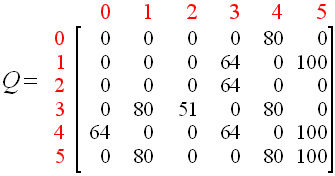This Is An Example Of A Fully Updated Q-Matrix.

In the beginning, all the values in the Q-table are set to zero; however, after every action, the table is updated so that the agent will remember what gives it the most optimal outcome.

In the Q-Learning equation above, Q is the Q-value you are trying to update based on the agent's action. R is the reward's value depending on the current state and action that the agent is in. So if you are in state 1 in the reward matrix and choose action 5, your reward would be 100.

Gamma represents the degree that the algorithm considers exploring new possibilities compared to choosing the same reward that has already been achieved. In this example, the gamma was set to 0.8.

This next part of the equation, max[Q(state prime, action)], is the highest Q-table value based on the already calculated state and action rewards. These values will all start at zero until they are updated using the formula. Once they are updated, you can plug them back into the equation to get more accurate results.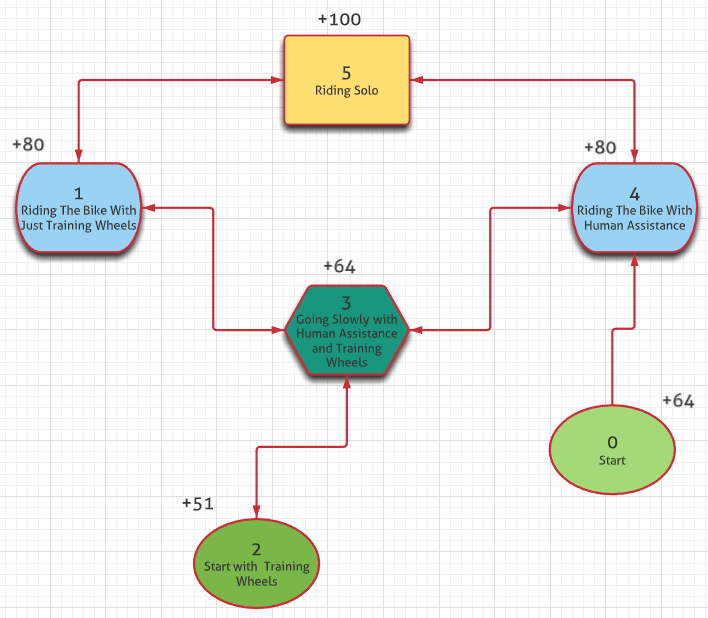Fully Updated Mind Map Showing Different Stages Of Riding The Bike

Using these two matrices, the agent can predict what actions will result in the highest reward. Once the Q-matrix is fully updated, it becomes the policy.

# Deep Q-Learning

## Why Use Deep Q-Learning

Q-learning can solve pretty basic problems; however, it has difficulties trying to solve more complicated tasks. If we want to have Self-Driving cars or AI that can achieve a super-human level in video games, we need to use Deep Q-Learning (DQN). DQN’s can solve for much more complex environments, and at the same time, generalize data much better.

If a normal Q-learning algorithm comes across a new scenario that it has never seen before, it will take a random action. In contrast, a DQN will find similarities between new and old scenarios to develop a much more precise action. This makes DQNs much better for tasks that require generalization.

If you wanted to create an AI algorithm that can beat humans in the game of Pong, you would need a huge Q-table. Assuming an image size of 124x64 in greyscale (with 256 grey scale levels), and 4 consecutive frames for each input, this amounts to 256 to the power of 31,744 (124x64x4) different rows in the Q-table. To put that into perspective, there is only 10 to the power of 82 atoms in the universe.

## What Exactly Are DQN’s

Deep Q-learning is the combination of using Deep Learning and Q-learning. Instead of tracking every single combination of actions in every state, we can use neural networks to solve for the expected Q-value. First, Convolutional Layers are used to find different data patterns, then Fully Connected Layers are used to output the expected Q-value for each available action.

# Methods That DQNs Use To Improve Performance

## Experience Replay

Experience Replay is a technique that allows Agents to optimize the amount of reward they get in a game. The way it works is that it saves the agent's experiences into the Replay Memory.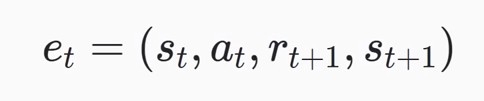This Is The Equation Used To Save Past Experiences

The Replay Memory is then what is used to train the network. The reason that this is done is that it is highly efficient. Neural networks tend to have catastrophic interference, which means that they can forget large amounts of data from past experiences. Experience Replay allows data from the past to be reused.

It is also more efficient because it allows the network to update the weights and biases in mini-batches(mini-batches are just smaller samples of the inputted data). This greatly increases computational efficiency because you do not have to take in all the data at once; instead, you can break it into smaller pieces.

Another key reason for using experience replay is that the network won’t always learn data from consecutive samples. The problem with learning from consecutive samples is that most AI-algorithms are based on the assumption of independent and identically distributed data(iid). This means that having data coming from a sequence would go against this iid and start to cause problems.

If you want to develop a better understanding of Experience Replay, go here.

## Fixed Target Networks

To understand Fixed Target Networks, we must first examine how Neural Networks calculate the Q-values. To do this, they use what is called the Policy Network. Before executing a backpropagation step, there are two different, forward passes.

In the first forward pass, the input is a random state you have saved in your replay memory, and the output is the current Q-values for every action in that state.

The second forward pass takes the state right after the randomly selected state and plugs it into a clone of the Policy Network. This is called the Target Network. The output of the Target Network is the target Q-value of all available actions.

The reason this is done is to calculate what’s referred to as the Loss (L). The Loss is the amount between the target value and the current value. The higher the loss, the worse the model is performing. To calculate the loss, you must subtract the Q-value based on the current State and Action from the target Q-value.

Here you can see that the part highlighted in red is the target Q-value, which is the same as in the Bellman equation. The Target Network is what is used to achieve that part of the loss function. The Q-Value then reduces it.

## Quickly Let’s Look At How The Neural Network Gets Updated

The process that is used is called Stochastic Gradient Descent. Once we have calculated the loss between the Target Q-values and the current Q-values, we can backpropagate through the network and update the weights and biases. The math behind this can get very complicated, so watch the video below if you want to look into it more.

## Back To The Fixed Target Network

It is called the Fixed Target Network because, instead of updating the weights for the Fixed Target Network every backpropagation step, you only update it every n amount of episodes. This allows the Policy Network to have a concrete target for a certain amount of time. After that amount of time is over, the Target Network copies the newly updated Policy Network's weights and biases, and the cycle repeats. This reduces a lot of instability in training, whereas if we were training both of these forward passes on the same Neural Network, it would be as if a cat is chasing its own tail.

The following table shows the effect Experience Replay and Target Network can have on the outcomes. The 0s represent when the Experience Replay or Target Network is in use, the x is when they are not.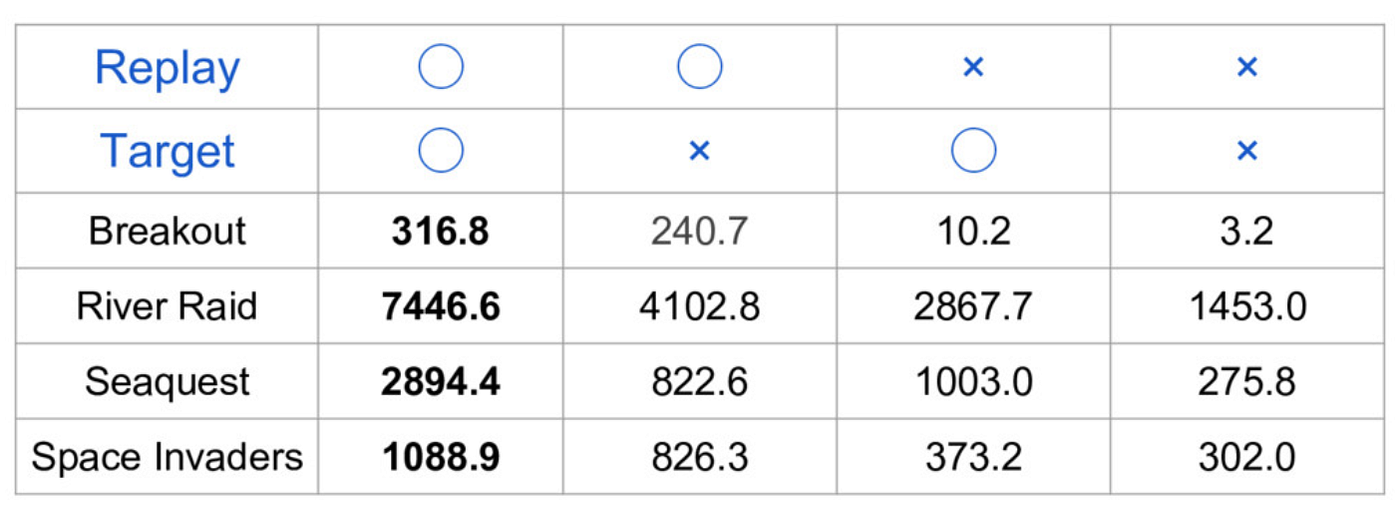Credit: Lex Fridman’s MIT 6.S091: Introduction to Deep Reinforcement Learning (Deep RL)

# Other Methods Of Training Deep Q-Networks

## Monte Carlo Tree Search

The Monte Carlo Tree Search(MCTS) algorithm has been used in many Deep Q-Learning projects, such as AlphaZero. It uses different branches to calculate the different possible outcomes of each series of events. The MCTS algorithm has four different components. The Selection, Expansion, Simulation, and Backpropagation.

1. Selection: During the selection phase, the algorithm chooses which path of actions it wants to go down. It decides this by choosing the node with the highest estimated value. To do this, it uses the following equation:

2. Expansion: After the algorithm has selected the node with the highest estimated value, and assuming that the game did not end after that action, it goes even further into the future and expands the tree according to the available actions.

3. Simulation: Once the tree is expanded, it simulates a random set of actions based on the policy until it reaches a terminal state (If the game is lost or won) or a simulation cap is reached. It then calculates the total value of this set of actions.

4. Backpropagation: Finally, after the algorithm computes the total values for each set of actions, it backpropagates through the network, updating all the weights and biases of each layer.

There are plenty of other Reinforcement Learning techniques, such as Policy Gradients, DDPGs, Quantile Regression DQNs, and many more!

AI is going to become the language of the future. Reinforcement Learning will play a huge role in its success. We will be able to create robots that can think for themselves, advanced self-driving cars, fully-automated factories, smart prosthetic limbs, and many more! If you want to learn more about any of these topics, I have included some links below.

## TL;DR

• Reinforcement Learning will be a big part of our future.
• The basics are it uses an Agent in an Environment(e), it then takes an Action(a) and is returned with a Reward(r) and a new State(s)
• Q-Learning uses a combination of two Matrices: The Reward Matrix and the Q-Matrix
• The problems with Q-Learning are that it has difficulties trying to solve more complex environments. It also has issues with generalization
• To solve this issue, we use a combination of Deep Learning and Q-Learning. This is called Deep Q-Learning.
• Deep Q-Learning takes 4 frames as input and then outputs the expected Q-value for each action
• Some techniques that DQNs use to improve performance are Experience Replay and Fixed Target Networks
• Experience Replay is when the machine stores data from past samples into what is called the Replay Memory. This data is then used to train the network
• Fixed Target Network is when the data is being trained; you separate two different, forward passes into two different networks. One is called the Policy Network; the other is called the Target Network.
• The Policy Network calculates the current Q-value based on the state and action chosen from the Replay Memory. Then the Target Network calculates the target Q-Value.
• These two Networks help calculate the loss, which is then used to backpropagate through the network and update the weights
• Another way of training Deep Q-Learning models is through the use of Monte Carlo Tree Search(MCTS)
• MCTS utilizes four different steps. Selection, Expansion, Simulation, and Backpropagation
• These four steps then help optimize the weights and biases to find an optimal policy. This is a very useful technique and is used in AlphaZero, which is one of the best Reinforcement Learning algorithms created
• There are plenty of other techniques used in Reinforcement learning such as Policy Gradients, DDPGs, Quantile Regression DQNs, etc

--

--